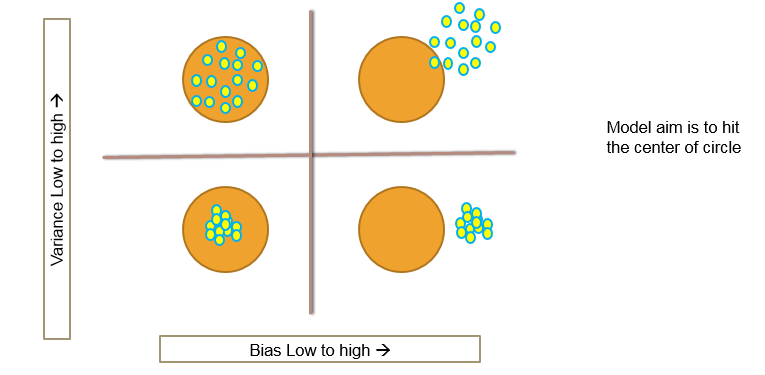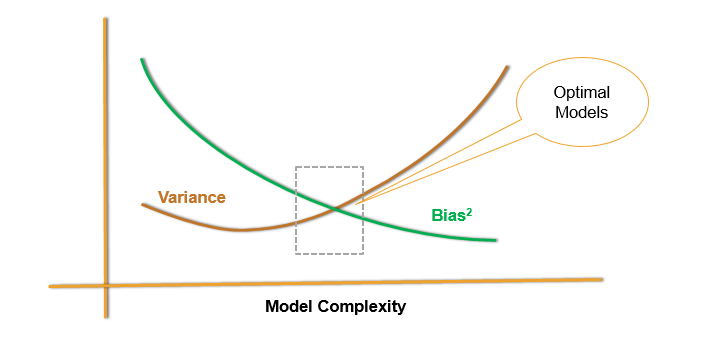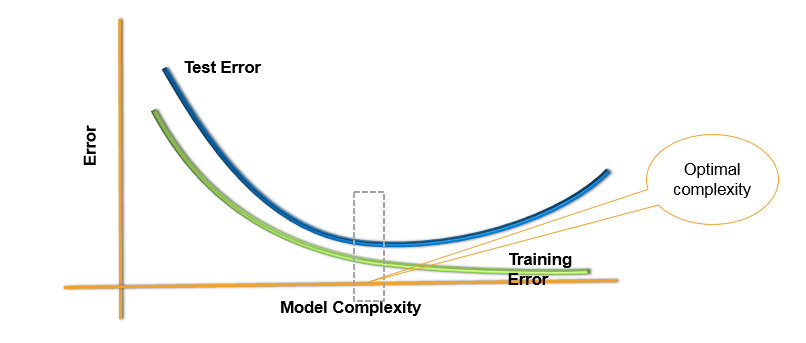• No products in the cart.

Sweet spot for Bias and Variance.
Link to the previous post : https://statinfer.com/204-4-8-problem-of-under-fitting/

Model Bias and Variance

• Over fitting
• Low Bias with High Variance
• Low training error – ‘Low Bias’
• High testing error
• Unstable model – ‘High Variance’
• The coefficients of the model change with small changes in the data
• Under fitting
• High Bias with low Variance
• High training error – ‘high Bias’
• testing error almost equal to training error
• Stable model – ‘Low Variance’
• The coefficients of the model doesn’t change with small changes in the dataThe Bias-Variance Decomposition

Y=f(X)+ϵ Var(ϵ)=σ2 SquaredError=E[(Yf^(x0))2|X=x0]

= σ2+[Ef^(x0)f(x0)]2+E[f^(x0)Ef^(x0)]2

= σ2+(Bias)2(f^(x0))+Var(f^(x0))

Overall Model Squared Error = Irreducible Error + $$Bias^2$$ + Variance

Bias-Variance Decomposition

• Overall error is made by bias and variance together.
• High bias low variance, Low bias and high variance, both are bad for the overall accuracy of the model.
• A good model need to have low bias and low variance or at least an optimal where both of them are jointly low.
• How to choose such optimal model. How to choose that optimal model complexity.Test and Training ErrorChoosing Optimal Model

• Unfortunately,there is no scientific method of choosing optimal model complexity that gives minimum test error.
• Training error is not a good estimate of the test error.
• There is always bias-variance tradeoff in choosing the appropriate complexity of the model.
• We can use cross validation methods, boot strapping and bagging to choose the optimal and consistent model.

The next post is about cross validation.

Link to the next post : https://statinfer.com/204-4-10-cross-validation/

0 responses on "204.4.9 Model-Bias Variance Tradeoff"